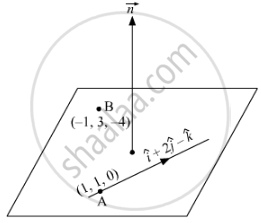# Find the Vector Equation of the Plane that Contains the Lines → R = ( ˆ I + ˆ J ) + λ ( ˆ I + 2 ˆ J − ˆ K ) and the Point (–1, 3, –4). Also, Find the Length of the Perpendicular Drawn - Mathematics

Sum

Find the vector equation of the plane that contains the lines vecr = (hat"i" + hat"j") + λ (hat"i" + 2hat"j" - hat"k") and the point (–1, 3, –4). Also, find the length of the perpendicular drawn from the point (2, 1, 4) to the plane thus obtained.

#### Solution

Let the vector equation of the required plane be  vec"r" . vec"n" = dThe plane contains the line vec"r" = hat"i" + hat"j" + λ (hat"i" + 2hat"j" - hat"k")

Since the plane passes through point A and B. So vec"n" will be parallel to vector vec"AB" xx (hat"i" + 2hat"j" - hat"k")

vec"AB" = vec"OB" -vec"OA"

= (-hat"i" + 3hat"j" - 4hat"k") (hat"i" + hat"j")

= -2hat"i" + 2hat"j" - 4hat"k"

vec"AB" xx (hat"i" + 2hat"j" - hat"k") = |(hat"i",hat"j",hat"k"),(1,2,-1),(-2,2, -4)|

= hat"i" (-8 + 2) - hat"j" (-4-2) + hat"k" (2+4)

= -6hat"i" + 6hat"j" + 6hat"k"

which is a normal vector to the plane.

So the equation of plane will be vec"r" . (-6hat"i" + 6hat"j" + 6hat"k") = d

∴ it passes through (1, 1, 0) so (hat"i" + hat"j"). (-6hat"i" + 6hat"j" + 6hat"k") = d or, d = 0

equation of plane is vecr . (-6hat"i" + 6hat"j" + 6hat"k") = 0

vecr (hat"i" - hat"j" -hat"k") = 0

in Cartesian plane,

(xhat"i" + yhat"j" + zhat"k") . (hat"i" - hat"j" -hat"k") = 0

x -y - z = 0

So, the perpendicular distance of the plane from the point (2, 1, 4) is   = |(2 -1-4)/sqrt(1^2 + (-1)^2 + (-1)^2)| = |(-3)/sqrt(3)| = sqrt(3) "unit".

Concept: Vector and Cartesian Equation of a Plane
Is there an error in this question or solution?